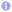PHP: Find number of weeks in a given month

The following function finds the number of weeks in a given month, assuming Monday as the first day of the week.

 Source code`<?phpfunction weeks_in_month(\$month, \$year) { // Start of month \$start = mktime(0, 0, 0, \$month, 1, \$year); // End of month \$end = mktime(0, 0, 0, \$month, date('t', \$start), \$year); // Start week \$start_week = date('W', \$start); // End week \$end_week = date('W', \$end);  if (\$end_week < \$start_week) { // Month wraps   return ((52 + \$end_week) - \$start_week) + 1; }  return (\$end_week - \$start_week) + 1;}`

1.thanks… Good code…

2.Hi,
I made a little fix to this function. Here is the code.

function weeks_in_month(\$month, \$year) {
// Start of month
\$start = mktime(0, 0, 0, \$month, 1, \$year);
// End of month
\$end = mktime(0, 0, 0, \$month, date(‘t’, \$start), \$year);
// Start week
\$start_week = date(‘W’, \$start);
// End week
\$end_week = date(‘W’, \$end);

if (\$end_week < \$start_week) { // Month wraps
//year has 52 weeks
\$weeksInYear = 52;
//but if leap year, it has 53 weeks
if(\$year % 4 == 0) {
\$weeksInYear = 53;
}
return ((\$weeksInYear + \$end_week) – \$start_week) + 1;
}

return (\$end_week – \$start_week) + 1;
}

My code includes leap year in calculations.

Best Regards,
Emil Jaszczuk

3.DStein Corp

Amazing, You Saved Me, Thank A Lot…